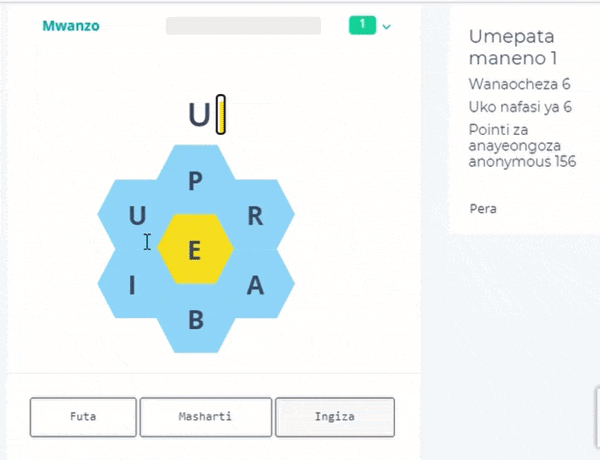Click the PDF icon to download the document## Large Integer Multiplication

12 years ago | 5070 reads
Mathematical operations are central part of many algorithms. In order to achieve faster computational speeds, these operations need to be efficient. Integer multiplication is one of those operations. Classrooms everywhere teach the classic method of multiplying each digit on one number with a digit of the other resulting in a complexity of O(n squared). Karatsuba introduced a method that required O(n power 1.54). His method used Divide &amp; Conquer. In this project, a similar algorithm is introduced. The algorithm uses the intuition of using Divide and Conquer as in the Karatsuba, but this time, squares of differences are utilized instead. Various optimizations are then discussed and implemented to improve its computational efficiency.

#### Chemsha bongo

NewHebu tuone utalaam wako wa kiswahili

Cheza ijumaa 09 Oktoba 2020. Unaweza jishindia Tsh. 30.000